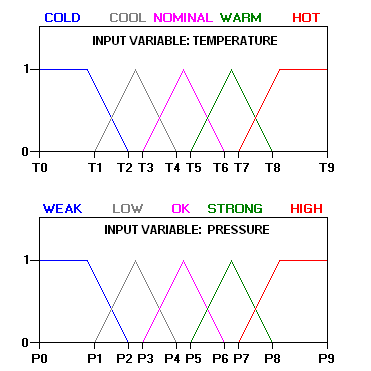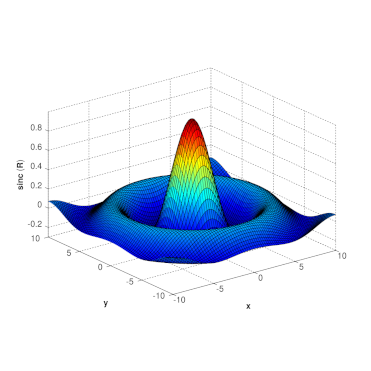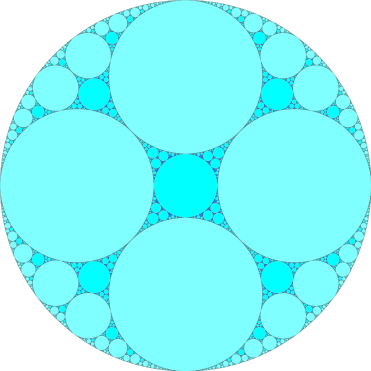# Conference scopes

The aim of the conference is to bring together theoreticians and practitioners working on fuzzy logic, fuzzy systems, soft computing and related areas. It will provide a platform for the exchange of ideas among scientists, engineers and students.

The topics addressed by the Conference cover all aspects of fuzzy logic and soft computing, namely:

• • Approximate reasoning
• • Clustering and classification
• • Cognitive modeling
• • Intelligent data analysis and data-mining
• • Data aggregation and fusion
• • Database management and querying
• • Theory and applications of decision-making
• • Forecasting and time series modeling
• • Fuzzy control
• • Theoretical foundations of fuzzy logic and fuzzy set theory
• • Imprecise probabilities and fuzzy methods in statistics
• • Image processing and computer vision
• • Information retrieval
• • Knowledge representation and knowledge engineering
• • Linguistic modelling
• • Machine learning
• • Natural language processing, generation and understanding
• • Neuro-fuzzy systems
• • Stochastic and fuzzy optimization
• • Possibility theory and applications
• • Rough sets theory
• • Semantic web
• • Uncertainty modeling

•••